Zephyr Pain Index

This risk metric captures the time it takes to regain investment losses, and the severity and frequency of those losses. Get a free spreadsheet with open VBA.

Market analysts quantify risk with several metrics; common examples are the downside deviation, volatility (i.e. standard deviation) and the maximum drawdown.

However, these quantities do not capture any information about time. How long has the security spent “underwater”? How long did the stock take to recover? Does the security often spend time trying to regain its losses?

To address the failures of these existing risk metrics, Zephyr Associates introduced the Pain Index in 2006. The Pain Index is defined this formula,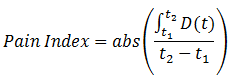where D(t) is the drawdown between time t1 and t2. This equation is essentially the area between the 0% line and the drawdown curve (between two dates) divided by the time span.

It’s clear from this equation that the Pain Index is similar to the Ulcer Index – both risk measures quantify investor stress.

Consider the chart below – it’s the historical drawdown for Apple (ticker: AAPL) between January 2000 and June 2014.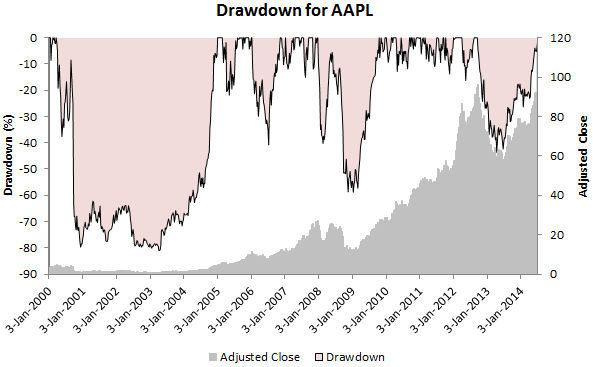The Pain Index would simply be the pink shaded area divided between any two dates by the time between the two dates.

For discrete set of drawdowns, the Pain Index is defined by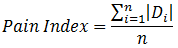From this formula, it’s clear that the the Pain Index is simply the average drawdown. It doesn’t matter if the drawdown is calculated on a day-by-day or month- by-month basis, as long as you average all the calculated drawdowns.

Of course, risk is just one side of the coin; risk needs to be traded off against return. For example, investors wouldn’t necessarily shy away from a volatile stock if the potential rewards are significant.

Similar in form to the Sharpe or Sortino Ratio, Zephyr Associates also introduced the Pain Ratio. This is equal to the effective return divided by the Pain Index.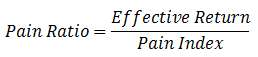Calculate the Zephyr Pain Index, CAGR and Maximum Drawdown in Excel

This Excel spreadsheet plots the drawdown and calculates the Pain Index. Just type in a ticker symbol, two dates and a frequency. After you click a button, Excel whirs aways and downloads historical stock prices from Yahoo.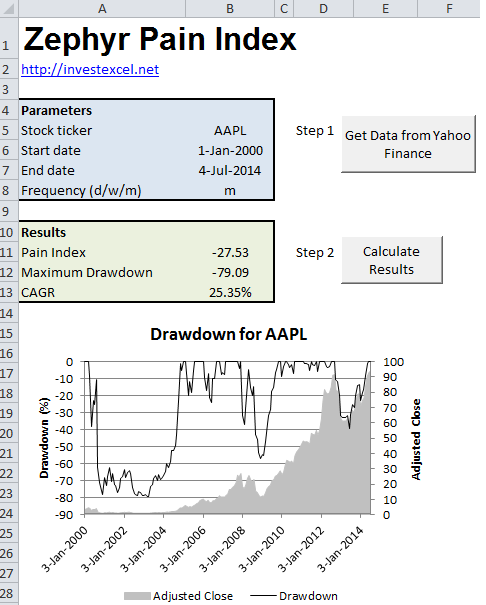It then plots the drawdown (based on the adjusted close price), calculates the Pain Index, compound annual growth rate and the maximum drawdown.

Everything is automated in VBA. The code is can be viewed, modified or altered – it’s not password protected! If you like this tool, please consider donating a few dollars, or linking to https://investexcel.net.